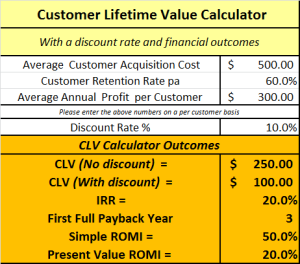# Financial Metrics for CLV

## Understanding the financial metrics for customer lifetime value

The full customer lifetime value calculator and the free Excel CLV template both provide a summary outcome for several related financial metrics. Please refer to the image below of the full CLV calculator. As you can see, at the bottom, there are multiple financial metrics listed, including:

• CLV (with and without a discount rate)
• IRR (internal rate of return)
• First full payback period
• Simple and present value return on marketing investment (ROMI)

Each these metrics are discussed under the image of the CLV calculator.### Financial Metrics for CLV

#### Customer lifetime value (no discount) = \$250

This is the overall profit contribution of the customer to the firm during their customer relationship period. It is calculated by the annual profit contribution multiplied by the number of years they are likely to be a customer less acquisition costs. Please refer to the simple customer lifetime value formula and examples.

#### Customer lifetime value (with discount) = \$100

This is the true customer lifetime value figure, as it takes into account the discount rate (and converts future cash flows into the equivalent cash flows in today’s money). For further information please refer to the main customer lifetime value formula.

#### Internal rate of return (IRR) = 20%

Internal rate of return (IRR) calculates the average return on the initial acquisition investment (which is \$500 average customer acquisition cost). Over time, the \$500 customer acquisition investment achieves an average annual return of 20%.

In simple terms, internal rate of return (IRR) is the average percentage return from customers over time (calculated on an annual basis).

#### First full year payback period = 3 years

A three year paybacks means that in year three, the company has made enough profit from the customer to have now fully repaid the initial acquisition cost. This calculation takes into account the customer retention rate (which is the probability of future cash flows being received from customers). Please refer to the full explanation of the customer lifetime value formula or to the video section.

#### ROMI = 50% (simple) and 20% (present value)

The first return on marketing investment (ROMI) figure of 50% s takes overall profit contribution (CLV = \$250) without consideration of the discount rate. In this case, the firm has made \$250 (CLV) for its initial acquisition investment of \$500 = \$250/\$500 = 50% ROMI.

The second ROMI calculation uses the true (discounted) figure of \$100. The ROMI then becomes \$100/\$500 = 20%.